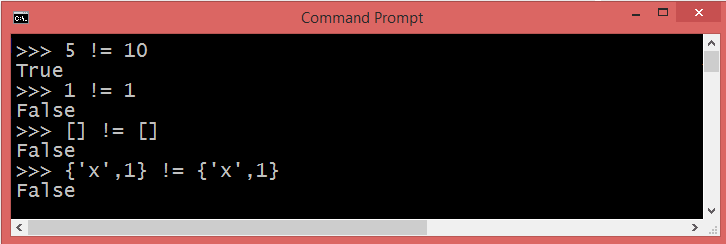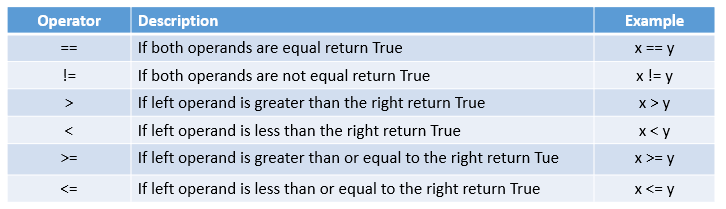Net-informations.com

# Not Equal operator in Python

You can use "!=" and "is not" for not equal operation in Python.

## Python != Operator

The python != ( not equal operator ) return True, if the values of the two Python operands given on each side of the operator are not equal, otherwise false .Python is dynamically, but strongly typed , and other statically typed languages would complain about comparing different types . So if the two variables have the same values but they are of different type, then not equal operator will return True.

## Python is not

The is operator is the object identity operator used to check if two objects in fact are the same and its negation is not : x is y is true if and only if x and y are the same object.

The above example will print "not equal" as x = 2 as assigned earlier.

## Python Comparison Operators

A comparison operator , also called python relational operator, compares the values on both sides of the operator to classify the relation between them as either true or false .net-informations.com (C) 2022    Founded by raps mk